# Neural Networks for Decision Boundary in Python!

One of the things I wanted to challenge myself with at the start of the year was how to use a neural network in python.

Artificial Neural Network (ANN) is an information-processing paradigm which is inspired by the brain it is often used in machine learning. It was initially proposed in the ’40s and there was some interest initially, but it disappeared soon due to ineffi­cient training algorithms used and the lack of computing power. However more recently they have been started to get used again, especially since the in­tro­duc­tion of au­toen­coders, con­vo­lu­tion­al nets, dropout reg­u­lar­iza­tion and other techniques that improve the performance of Neural Networks significantly.

Neural networks are formed by neurones that are connected to each other which send each other signals. If the number of signals a neurone received is over a threshold, it then sends a signal to other neurones it is connected to. In the general case, the con­nec­tions can be between any neurones, even to themselves, but it sometimes gets pretty hard to train them, so in most cases, there are several re­stric­tions to them.

In the case of the multi-layer perceptron, neurones are arranged in layers, and each neurone sends signals only to the next neurones in the following layer. The first layer consists of the input data, while the last layer is called the output layer and contains the predicted values. All the neurones are connected by what we call synapse.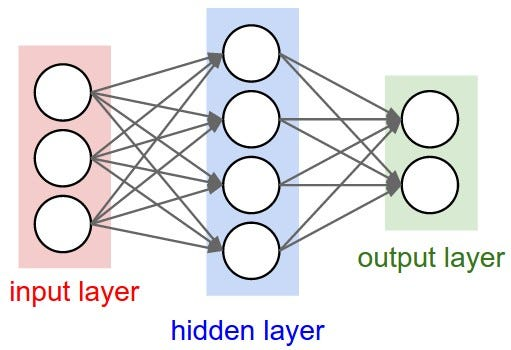A neural network diagram

Instead of using a hard threshold to decide whether to send a signal or not, neural networks use sigmoid functions.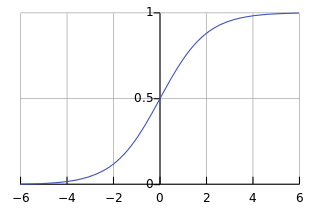How a sigmoid function looks like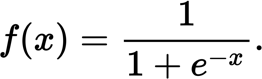The Equation.

We used the Sigmoid curve to calculate the output of the neurone.

The most common ways how you train a Neural network has two phases:

1. A forward pass, in which the training data is run through the network to obtain its output called as feed forward.
2. A backwards pass, in which, starting from the output, the errors for each neurone are calculated and then used to adjust the weight of the network called as backpropogation.

In this post, we will implement a simple 3-layer neural network. Which will replace logistic Regression for drawing a decision boundary which can show how powerful neural networks can be!

Here I’m assuming that you are familiar with basic Machine Learning concepts, e.g. you know what classification and regularization are. Ideally, you also know a bit about how optimization techniques like how does gradient descent work.

So we will not go too much into math today and we will use some machine learning libraries in python

So now comes to the Implementation

### Logistic Regression

Lets implement a decision boundary in Logistic Regression first.

So let’s train a Logistic Regression classifier. It’s input will be the x- and y-values of a dataset and the output the predicted class (0 or 1(for the decision boundary)). To make our life easy we use the library called scikit-learn..

So lets train the logistic regression classifier and plot it.

In this code below are using a readymade dataset

The imports we will need for all the code are:

`# Package imports import matplotlib.pyplot as plt import numpy as np import sklearn import sklearn.datasets import sklearn.linear_model import matplotlib`

So now lets show out plots for that we need to type in

`# Generate a dataset and plot itnp.random.seed(0)X, y = sklearn.datasets.make_moons(200, noise=0.20)plt.scatter(X[:,0], X[:,1], s=40, c=y, cmap=plt.cm.Spectral)plt.show()`

This will give you the plot of the dataset: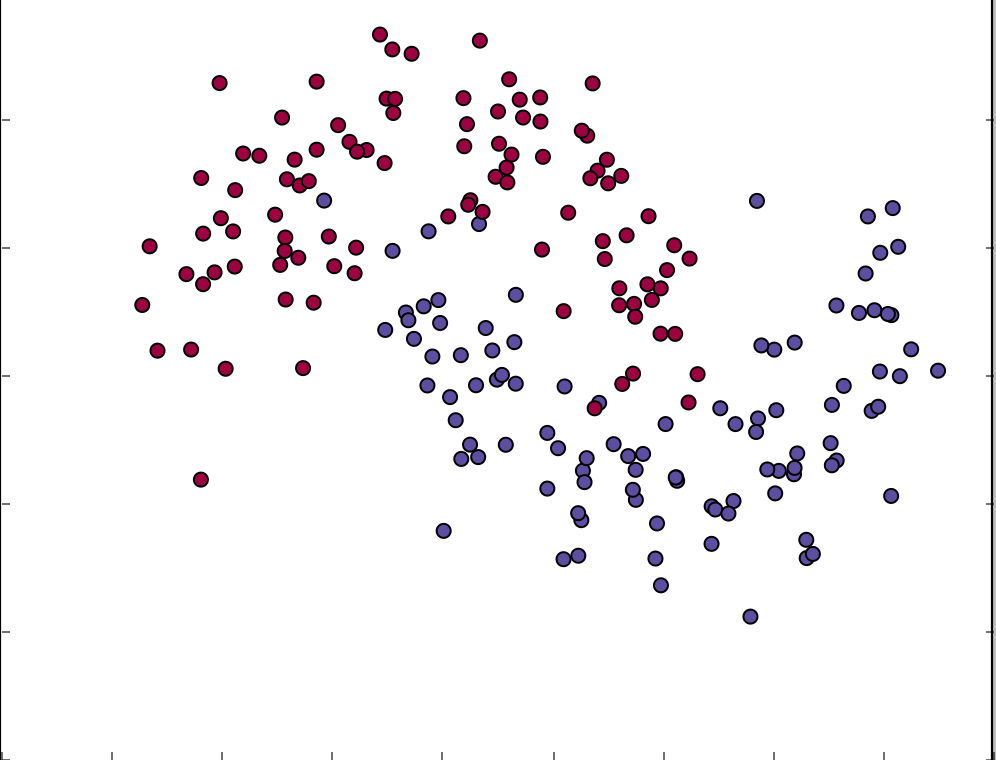Plot of the dataset

Now we will train our `logistic regression classifier` on it.

This is all with the scikit-learn library

``# Train the logistic regression classifier``
``clf =` `sklearn.linear_model.LogisticRegressionCV()``
``clf.fit(X, y)``
``# Plot the decision boundary (the method is in the main code link provided in the end)``
``plot_decision_boundary(lambda` `x: clf.predict(x))``
``plt.title("Logistic Regression")``

This would end up with this :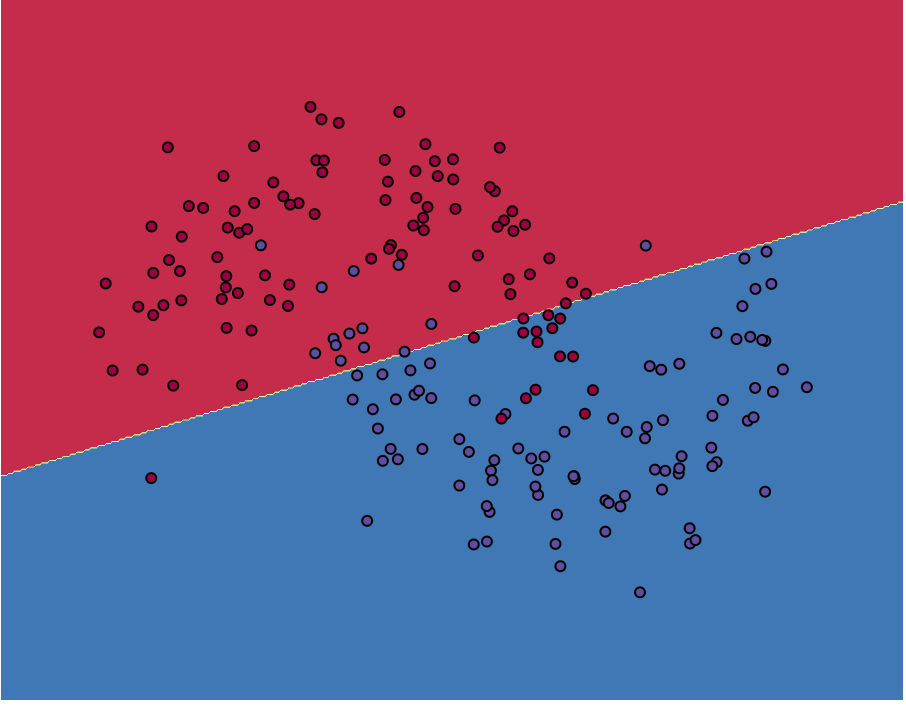Decision Boundary with logistic Regression.

The Logistic Regression classifier separates the data as good as it can using a straight line, but what if we want to make it more accurate?

So we would now try this same with Neural networks and you will see how better it gets!.

### TRAINING A NEURAL NETWORK

Let’s now build a 3-layer neural network

The picture below is how our 3 layer Neural Network will look like.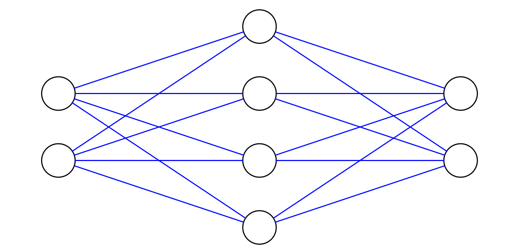Diagram of the 3 layer Neural Network.

To choose the dimensionality (the number of nodes) of the hidden layer you can say the more nodes we put into the hidden layer the more complex functions we can fit into it.

But High dimensionality can come at the cost of more computational power is needed to make predictions and learn the parameters of the network. A big number of parameters can lead to overfitting.

Choosing the size of the hidden layer always depends on the specific problem and is more of an art than science. You will see later in this blog post how the number of hidden layer affect the output.

• The neural network will have one input layer, one hidden layer, and one output layer.
• The number of nodes in the input layer is determined by the dimensionality of our data,
• The number of nodes in the output layer is determined by the number of classes we have. (in this case 2) as we have 0 and 1.

Now we also need to pick an activation function for our hidden layer. The activation functions transform the inputs of the layer into its outputs. A nonlinear function can allow us to fit the nonlinear hypothesis.

The Common choices for activation functions are tanh, the sigmoid function, or ReLUs.

For now, we will be using tanh.

As we want out the network to output probabilities the activation function for our output layer will be softmax, which is a simple way to convert raw scores to probabilities. If you’re familiar with the logistic function you can think of softmax as its generalization to multiple classes.

### How does our Neural Network makes predictions?

Our network makes predictions using forward propagation as it is a feedforward neural network. that minimise the error in our training data. But how do we define the error? We call the function that measures our error the loss function. A common choice with the softmax output is the cross-entropy loss.

### Learning the Parameters

Learning the parameters for our network means finding parameters that minimize the error on our training data. But how do we define the error? We call the function that measures our error the loss function. A common choice with the softmax output is the cross-entropy loss.

### Implementation with Neural Networks

We start by defining some useful variables and parameters for gradient descent: (They are handpicked)

``num_examples =` `len(X) # the training set size``
``nn_input_dim =` `2` `# dimension of the input layer``
``nn_output_dim =` `2` `# dimension of the output layer``
``# Gradient descent parameters``
``epsilon =` `0.01` `# the learning rate for gradient descent``
``reg_lambda =` `0.01` `# the strength of regularization``

Now lets define a loss function to evaluate how our model is doing.

``def` `calculate_loss(model):``

Now we also have a helper function to predict an output (0 or 1)

``def` `predict(model, x):``

Finally, here comes the function to train our Neural Network. It implements batch gradient descent using the backpropagation derivates we found above.

So Now we will define a function called build_model

``def` `build_model(nn_hdim, num_passes=20000, print_loss=False):``

### A NETWORK WITH A HIDDEN LAYER OF SIZE 3

Let’s see what happens if we train a network with a hidden layer size of 3.

``# Build a model with a 3-dimensional hidden layer``
``model =` `build_model(3, print_loss=True)``
``# Plot the decision boundary``
``plot_decision_boundary(lambda` `x: predict(model, x))``
``plt.title("Decision Boundary for hidden layer size 3")``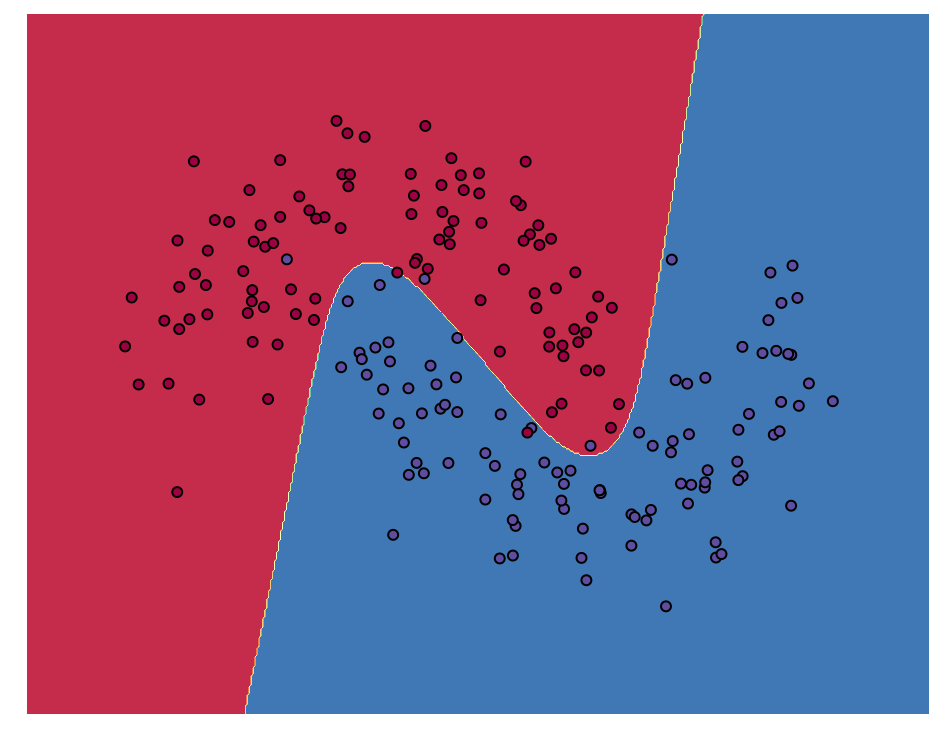Decision Boundary if hidden layer size is 3

Now for many cases.

We can see that a hidden layer of low dimensionality nicely captures the general trend of our data. Higher dimensionalities can lead to overfitting as they are memorising the data but they are supposed to do which is fitting the general shape. If we were to evaluate our model on a separate test set (which you should!) the model with a smaller hidden layer size would likely perform better because of better generalization. But we can counteract overfitting with stronger regularization, but picking the correct size for hidden layer is a much more “economical” solution.

As you can see that the more they are getting trained the better results you are getting.

As we can see the hidden layer with low dimensionalities are the ones which nicely get the general trend if it gets a bit higher it leads to overfitting.

The model with smaller hidden layer size would likely perform better because it generalizes better. We could counteract overfitting with stronger regularization, but picking the correct size for hidden layer is a much more “situation based” solution.

The full code is below and in an iPython notebook

If you enjoyed it please leave a 💚 on this post.

See you next time :)